# Deep Learning for Image-Like Data

From Probabilistic Deep Learning with Python by Oliver Dürr, Beate Sick, and Elvis Murina

This article discusses using deep learning for data that act like images.

__________________________________________________________________

Take 37% off Probabilistic Deep Learning with Python by entering fccdurr into the discount code box at checkout at manning.com.
__________________________________________________________________

## Using a fully connected NN to classify images

Let’s now use your new skills to build a larger network and see how it performs on the task of classifying handwritten digits. Different scientific disciplines have different model systems that benchmark their methods. Molecular biologists use a worm called C. Elegance, people doing social network analysis use the Zachary Karate Club, and finally, people doing DL use the famous MNIST digit data set. This benchmark dataset consists of 70,000 handwritten digits and it’s available from http://yann.lecun.com/exdb/mnist/. The images all have 28 x 28 pixels and are gray scaled with values between 0 and 255. The first four images of the dataset are displayed in Figure 1.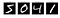Figure 1 The first four digits of the MNIST data set — the standard data set used for benchmarking NN for images classification

This data set is well-known in the machine learning community. If you develop a novel algorithm for image classification, you usually also report its performance on the MNIST data set. For a fair comparison, there’s a standard split of the data: 60,000 of the images are used for training the network, and 10,000 are used for testing. In Keras, you can download the whole data set with a single line (see listing 3) and also the companion MNIST notebook for this section (on which you can work later) at https://github.com/tensorchiefs/dl_book/blob/master/chapter_02/nb_ch02_02a.ipynb.

Simple neural networks can’t deal with 2D images but need a 1D input vector. Instead of feeding the 28 x 28 images directly, you first flatten the image into a vector of size 28 * 28 = 784. The output should indicate whether the input image is one of the digits zero through nine. More precisely, you want to model the probability that the network thinks that a given input image is a certain digit. For this the output layer has ten neurons (one for each digit). You again use the activation function, softmax, to ensure that the computed outputs can be interpreted as probabilities, which are numbers between zero and one, adding up to one. For this example, we also include hidden layers. Figure 2 shows a simplified version of the network and the definition of the corresponding model in Keras is shown in listing 4.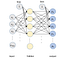Figure 2: A fully connected NN with two hidden layers. For the MNIST example, the input layer has 784 values for the 28 x 28 pixels and the output layer out of ten nodes for the ten classes.

`from keras.datasets import mnist (x_train, y_train), (x_test, y_test) = mnist.load_data() #A     X_train = x_train[0:50000] / 255 #C Y_train = y_train[0:50000] #D Y_train = keras.utils.to_categorical(Y_train,10) #E X_val=x_train[50000:60000] / 255 #F ...`

# A Loads the MNIST training (60,000 images) and test set

# C Uses 50,000 for training and divides by 255; values are in the range 0–1

# D Stores the labels as integers from zero to nine

# F We do the same with the validation set.

Note that we don’t use the testset for this listing.

Also, where we store the labels for the y_train, for the network, we transform those to categorical data of length ten to match the output. A 1 is translated as (0,1,0,0,0,0,0,0,0,0). This is called one-hot encoding.

Listing 4. Definition of an fcNN for the MNIST data

`model = Sequential()   model.add(Dense(100, batch_input_shape=(None, 784), #A                                   activation=’sigmoid’)) model.add(Dense(50, activation='’sigmoid’'))  #B model.add(Dense(10, activation='softmax')) #C     model.compile(loss='categorical_crossentropy',               optimizer='adam', # D                             metrics=['accuracy']) #D2   history=model.fit(X_train_flat, Y_train,                    batch_size=128,                   epochs=10,                   validation_data=(X_val_flat, Y_val)                   )`

#A The first hidden layer with one hundred neurons, connected to the input size 28*28 pixels

#B A second dense layer with fifty neurons

#C The third layer connecting to the ten output neurons

#D Uses a different optimizer then the SGD, which is faster

#D2 Tracks the accuracy (fraction of correctly classified training and validation examples) during the training

Now open the MNIST notebook https://github.com/tensorchiefs/dl_book/blob/master/chapter_02/nb_ch02_02a.ipynb run it and try to understand the code.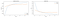Figure 3 The increase of the accuracy training (left) and the decrease of the loss (right) during the different training steps

When looking at the course of the loss curves over the number of iterations (figure 3), you can observe that the model fits the data. The performance of the trained fcNN on the validation set’s around 97%, which isn’t bad, but the state of the art’s about 99%.

Play the DL game and stack more layers. Another trick which is often used is to replace the sigmoid activation function in the hidden layers with something easier, called ReLU. ReLU stands for Rectified Linear Unit and it’s quite a mouthful for what it does. It clamps values smaller than zero to zero and leaves values larger than zero as they are (see figure 4). To change the activation in Keras, exchange sigmoid with relu. If you like, change the activation function in the notebook https://github.com/tensorchiefs/dl_book/blob/master/chapter_02/nb_ch02_02a.ipynb.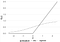Figure 4 A comparison between the ReLU and the sigmoid activation functions

Let’s do a small experiment and investigate what happens if you shuffle the pixel values before you feed them into the network. Figure 5 shows the same digits as in Figure 1, but this time randomly shuffled.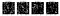Figure 5: The same digits (5,0,4,1) as in figure 1 after shuffling the pixels

For each image, the pixels have been shuffled the same way. You’d have a hard time telling the right digit even after seeing thousands of training examples. Can a network still learn to recognize the digits?

Try it out and play with the code in the MNIST notebook https://github.com/tensorchiefs/dl_book/blob/master/chapter_02/nb_ch02_02.ipynb. What do you observe?

NOTE: Only follow the notebook until you reach the section “CNN as a classification model for MNIST data.” We’ll look at CNNs later and will then revisit the notebook.

You’ll probably reach the same accuracy (within statistical fluctuations) as with the original images. This might come as a surprise at first. Looking at the network architecture of an fcNN, the order of the input doesn’t matter. Because the network has no concept of nearby pixels, there’s nothing like a neighborhood. People also call fcNN permutation invariant NN because its performance doesn’t depend on whether the data is permuted (shuffled). Real image data isn’t permutation invariant, and nearby pixels tend to have similar values. If you shuffle images, people will have a problem recognizing those. Moreover, two images showing the same digit don’t need to show the same pixel values. You can move (translate) the image a bit, and it still shows the same object.

The fact that humans are great in visual tasks but have problems with shuffled images indicates that the evolution has found ways to take advantage of the special properties of image data. Although FC networks are good for spreadsheet-like data, where the order of the columns doesn’t matter, there are better architectures when the order or the spatial alignment matters, like convolutional NNs. In principle, fcNN can be used for images, but you need many layers and huge training data sets that allow the network to learn that nearby pixels tend to have the same values and that images are translation invariant.

## 2D convolutional NNs for image-like data

Fully connected NNs with even a single hidden layer can represent any function, but quickly get too big and contain many parameters that you usually don’t have enough data to fit them. Much of the progress in DL has been around creating different architectures that more efficiently exploit the structure of the data. For image data, one such architecture is convolutional NNs.

For the example of an fcNN with only one hidden layer (see figure 8), we discussed that the number of neurons in the hidden layer can be seen as the number of new features which are constructed from the input. This implies that you need a large number of neurons in the hidden layer if you want to tackle a complex problem, but the more neurons you have in the hidden layer, the more parameters you need to learn, and the more training data you need. Stacking layers lets the model learn task-specific features in a hierarchical manner. This approach needs fewer parameters than a fcNN to construct complex features and is less data hungry.

You learned in the last section that you get more out of an fcNN if you add more hidden layers. Going deep is a great trick to enhance the performance of NNs, and it gave DL its name. You also learned that an fcNN ignores the fact that the neighboring structure of pixels in an image matter. This suggests that there might be a better NN architecture to analyze image data. And indeed, the success of DL in the field of computer vision wouldn’t have been possible without some additional architectural tricks that exploit the knowledge about the local structure of image data.

The most important ingredient to tailor a NN for locally correlated data such as image data is the convolutional layers. We explain how a convolutional layer works. NNs that consist mainly of convolutional layers are called convolutional neural networks (CNNs) and have an extremely broad range of applications including the following:

• Image classification, such as discriminating a truck from a road sign
• Video data prediction, predicting radar images for weather forecasting, for example
• Quality control in production lines based on image or video data
• Classification and detection of different tumors in histopathological slices
• Segmentation of different objects in an image

## Main ideas in a CNN architecture

Let’s focus on image data and discuss a specialized NN architecture that takes into account the highly local structure within an image (see figure 6). This architecture, CNN, was used in 2012 by Alex Krizhevsky in the internationally renowned ImageNet competition, which brought the breakthrough of DL into the field of computer vision.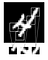Figure 6: An image can be broken into local patterns such as edges, textures.

We’ll now dive into the architecture of CNNs and discuss how they got their name. Let’s look at the main idea of a CNN: instead of connecting all neurons between two successive layers, only a small patch of neighboring pixels is connected to a neuron in the next layer (see figure 7). With this simple trick, the network architecture has the local structure of images built in. This trick also reduces the number of weights in the NN. If you only consider small patches of, for example, 3 x 3 pixels as a local pattern (see figure 8) which is connected to a neuron in the next layer, then you’ve only 3 ∙ 3 + 1 = 10 weights to learn for the weighted sum z = x1 ∙ w1 + x1 ∙ w1 + ∙∙∙+x9 ∙ w9 + b, which is the input to the next neuron.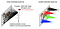Figure 7: Connectivity between the input image and a neuron in the first hidden layer for (a) an fcNN or (b) a convolutional NN. The bias term’s ignored in this representation.

## >

If you’ve experience with classical image analysis, then you know that the idea isn’t new at all. What you’re doing here’s called convolution.

Have a look at figure 8, where you see a small image with 6 x 6 pixels and a 3 x 3 kernel with predefined weights. You can slide the kernel over the image by taking steps of one pixel (called stride = 1). At each position, you compute the element-wise multiplication of the image pixel and the overlaid kernel weights. You then add theses values to get the weighted sum z = x1 ∙ w1 + x1 ∙ w1 + ∙∙∙+xk ∙ wk + b, where k is the number of pixels connected to each neuron and b a bias term. The computed value z is a single element of the output matrix. After shifting the kernel to the next position over the image, you can compute the next output value z. The resulting output is called an activation map or feature map.

In the example in figure 18, we start with a 6 x 6 image, convolve it with a 3 x 3 kernel, and receive a 4 x 4 activation map. Sliding a kernel over an image and requiring that the whole kernel is at each position completely within the image, yields to an activation map with reduced dimensions. For example, if you’ve a 3 x 3 kernel on all sides, one pixel is knocked off in the resulting activation map; in case of a 5 x 5 kernel, even two pixels. If you want to have the same dimension after applying the convolution, you can use a zero padding of the input image (called `padding='same'`, the argument of the convolution layer in listing 5; if you don’t want zero padding, the argument’s `padding='valid'`).

In CNNs, the kernel weights are learned. Because you use the same kernel at each position, you’ve shared weights, and you only need to learn (in our example) 3 ∙ 3 = 9 weights to compute a whole activation map. Usually a bias term is also included in case there are ten weights to learn. To interactively apply different kernels to a real image, see http://setosa.io/ev/image-kernels/.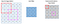Figure 18: Convolution of a 6 x 6 grey value image with a 3 x 3 kernel with stride 1 and without padding yield as output a 4 x 4 feature map. The kernel is applied at all sixteen possible positions to determine the sixteen values of the activation map. Two possible kernel positions are marked in the input with a thick solid and dashed border. The respective positions of the resulting pixels in the activation map are also marked with a thick solid and dashed border. The resulting values are computed by multiplying the pixel values with the overlaid kernel values and adding all terms (bias is assumed to be zero).

What can the values in an activation map tell you? If you apply a kernel to all possible positions within the image, you get only a high signal where the underlying image shows the pattern of the kernel. Assembling the outputs z to an image yields a map that shows at which positions in the image the kernel pattern appears. This is the reason why the resulting image’s often called feature map or activation map.

Each neuron in the same activation map has the same number of input connections, and the connecting weights are also the same. (You’ll see soon that in real applications, more than one kernel’s used.) Each neuron is connected to a different patch of the input (previous layer), meaning that each neuron within the same feature map’s looking for the same pattern but at different positions of the input. In figure 9, this concept is demonstrated for an abstract image that consists of rectangular areas where a kernel with a vertical edge pattern is applied. In image manipulation, this technique is used, for example, to enhance the edges of an image or to blur it. Visit again http://setosa.io/ev/image-kernels/ to get some feel for the effect of different kernels on a more complex image.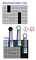Figure 9: Convolution of a 3 x 3 kernel with a weight pattern that resembles a vertical edge with an image consisting of squared areas results in a feature map that highlights the positions of vertical edges in the input image.

In figure 9, you can see the vertical-edge-kernel in three positions of the image. At the leftmost position, there’s a vertical edge in the image and the result’s a high value (shown as dark grey pixels in the activation map). At the two other positions, there’s no vertical edge in the image and the resulting values are much smaller (shown as lighter grey pixels in the activation map).

## A minimal CNN for edge lovers

Let’s imagine an art lover who gets excited if an image contains vertical edges. Your task is to predict for a set of striped images if the art lover likes them. Some of the images in the set have horizontal edges and some vertical edges. To identify the images with vertical stripes, a vertical-edge detection model would be great. For this purpose, you might want to do something similar to that depicted in figure 9 and perform a convolution of a predefined vertical-edge-filter, using the maximal value in the resulting feature map as a score that indicates if the art lover will like the image.

Using a predefined kernel for convolution is often done in traditional image analysis when the feature of interest is known and can be described as a local pattern. In such a situation, it’d be rather silly not to use this traditional image analysis approach. Let’s pretend you don’t know that the art lover likes vertical edges, and you only have a list of images that they like and dislike. You want to learn the values for the weights within the kernel which should be used for convolution. Figure 10 shows the corresponding network architecture, where the size of the kernel is 5 x 5. The resulting hidden layer is a feature map.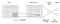Figure 10: A CNN with only one hidden layer consisting of one feature map. As a pooled value, the maximum of all values within the feature map is taken. A dense layer is added to determine the probability for two possible class labels in the output.

To check if this feature map indicates that the image contains the kernel pattern, you take the maximum value of the feature map. From this value, you want to predict the probability that the art lover likes the image. You already know how to do that: you add a single, fully connected layer with two output nodes and use softmax activation to ensure that the two output values can be taken as probabilities for the two classes (art lover likes the image; art lover doesn’t like the image), which adds up to one. This small CNN network (the feature map in the first hidden layer) results from the convolution of the image with a kernel. The classification is done in the fully connected part shown on the right side in figure 10. This architecture is probably one of the smallest possible CNNs one can think of.

To model image data with TensorFlow and Keras, you need to create 4D tensors with the form:

## (batch, height, width, channels)

The batch dimension corresponds to the number of images in one batch. The next two elements define the height and width of the image in units of pixels. The last dimension defines the number of channels (a typical RGB color image has three channels. This means that a batch of 128 color images, each having 256 rows and columns, could be stored in a tensor of shape (128, 256, 256, 3).

Setting up, training, and evaluating the CNN model can be done in a few lines of Keras code (see listing 5). The only thing you need is a data set of images with horizontal or vertical stripes and a corresponding class label. This can be easily simulated.

Open the edge lovers notebook https://github.com/tensorchiefs/dl_book/blob/master/chapter_02/nb_ch02_03.ipynb and follow the instructions there. You’ll simulate the image data and fit the model. Check out which kernel weights are learned and if they form a vertical edge, and if this is reproducible if you do the training over and over again. Investigate the impact of the activation function and the pooling method.

Listing 5. Edge lover CNN

`<  model = Sequential()   model.add(Convolution2D(1,(5,5),padding='same',input_shape=(pixel,pixel,1))) #A model.add(Activation('linear')) #B # take the max over all values in the activation map model.add(MaxPooling2D(pool_size=(pixel,pixel))) #C model.add(Flatten()) #D model.add(Dense(2)) #E model.add(Activation('softmax'))#F   # compile model and initialize weights model.compile(loss='categorical_crossentropy',               optimizer='adam',               metrics=['accuracy'])   # train the model history=model.fit(X_train, Y_train,                   validation_data=(X_val,Y_val),                   batch_size=64,                   epochs=15,                   verbose=1,                   shuffle=True)`

#A Uses a convolutional layer with one kernel of the size 5×5 with the same padding

#B Adds a linear activation function (passes all values through)

#C The MaxPooling layer extracts the maximal value of the feature map

#D Flattens the output of the previous layer to make it a vector

#E A dense layer with two neurons predicts the probabilities of two labels

#F Adds a softmax activation function to compute the probability for the two classes

In the listing, using a convolutional layer with `padding='same'` means that the output feature map has the same size as the input image.

In your experiments with the edge lover notebook https://github.com/tensorchiefs/dl_book/blob/master/chapter_02/nb_ch02_03.ipynb, you’ve probably seen that a vertical edge kernel isn’t always learned but sometimes also a horizontal edge kernel’s learned instead. This is perfectly fine because the data set consists of images with either horizontal or vertical edges, and the task is only to discriminate between horizontal and vertical edges. Finding no horizontal edges indicates that the image contains vertical edges.

In this edge-lover example, it probably makes no difference if you use a predefined kernel or learn the weights of the kernel, but in a more realistic application, the best discriminating pattern’s sometimes hard to predefine, and learning the optimal kernel weights is a great advantage of CNNs.

## Biological inspiration for a CNN architecture

The edge lover example was only a toy, and you might think that there’s certainly no edge-loving neuron in a real brain. The opposite is true! The visual cortex in the brains of humans and animals indeed has such edge-loving neurons. Two biologists, Hubel and Wiesel, even received the Nobel prize for discovering this in 1981. The way they found this is quite interesting. And, as is often in research, there was a great deal of luck involved.

In the late 1950s, Hubel and Wiesel wanted to investigate the correlation of the neuronal activity due to stimuli in the visual cortex of a cat. For this purpose, they anesthetized a cat and projected some images on a screen in front of it. They picked a single neuron to measure the electric signal (see figure 11). The experiment didn’t seem to work because they couldn’t observe the neuron firing while presenting different images to the cat. They changed the slides in the projector to those of an increasingly higher frequency. Finally, they shook the projector because a slide got stuck and then the neuron started to fire. In this manner, they discovered that neurons in different positions in the visual cortex are activated if edges with different orientations slide over the retina of the cat’s eye.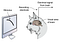Figure 11: Setup of the experiment of Hubel and Wiesel in which they discovered neurons in the visual cortex that responded when moving edges were shown to the cat.

Brain research continued to develop and now it’s widely known that in the area of the brain where Hubel and Wiesel did their experiments (called the V1 region), all neurons respond to rather simple forms of stimuli on different areas of the retina. This isn’t only true for cats, but also for other animals and humans. It’s also known that neurons in other regions of the brain (called V2, V4, and IT) respond to increasingly complex visual stimuli like, for example, a whole face (see figure 12). Research has shown that a neurons’ signal is transmitted from region to region. Also, only parts of the neurons in one region of the brain are connected with the neurons in the next region. Via the connections of the neurons, the activation of different neurons is combined in a hierarchical way that allows the neurons to respond on increasingly larger regions in the retina and to more and more complex visual stimuli (see figure 12).

NOTE: You’ll see soon that the architecture of deeper CNNs are loosely inspired by this hierarchical detection of complex structures from simple structures. The analogy shouldn’t be overstressed; the brain isn’t wired up to form a CNN.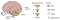Figure 12: Organization of the visual cortex in a brain, where neurons in different regions respond to an increasingly larger receptive field and more and more complex stimuli.

## Building and understanding a CNN

More realistic image classification tasks can’t be tackled by such a simple CNN architecture, such as that depicted in figure 10, which only learns to detect one local image pattern like an edge. Even simple image classification tasks, like discriminating between the ten digits in the MNIST data set, require learning lots of more complex image features. You can probably already guess how to do that: going deep is the main secret. But before going deep, you need to go broad and add more kernels to the first layer.

Each kernel can learn another set of weights, and for each kernel you get another activation map in the hidden layer (see figure 13). If the input has not only one but d channels, then the kernel also needs to have d channels to compute an activation map. For color images, you’ve d = 3 for (red, green, blue), and a valid kernel could be one which is active for a vertical edge in the green channel and horizontal edges in the blue and red channel. The kernel matrix again defines the weights for the weighted sum, which determines the input to the neuron in the respective position of the activation map.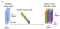Figure 13: Convolution of the input image with six different kernels results in six activation maps. If the input image has only one channel (a), then each kernel has also only one channel. If the input image has three channels (b), then each filter has also three channels.

Now let’s talk about analogies between in fcNN and CNNs. For each neuron in an fcNN, a new set of weights is learnt that combines the input of the former layer to a new value that can be seen as a feature of the image (see, for example, figure 8). In an fcNN, you can go deep by adding layers where all neurons of one layer are connected to all neurons in the next layer. In this sense, the number of kernel sets or activation maps in a CNN correspond to the number of neurons in one layer of an fcNN. If you want to go deep in a CNN, you need to add more convolutional layers. This means you learn kernels which are again applied to the stack of activation maps of the previous layers.

Figure 25 illustrates this principle. In that figure, you see a CNN with three convolutional layers. The convolution over a stack of activation maps isn’t different than the convolution with an input of several channels. In figure 13, only six activation maps are generated from a 3-channel input image. Learning only six kernels isn’t common. A typical number to learn is thirty-two kernels in the first layer and even more kernels (often the number of kernels doubles when moving from layer to layer). To reduce the number of weights in a CNN, it’s also common to downsample the activation maps before doing the next round of convolution. This is often done by replacing a 2 x 2 patch of neurons in an activation map with the maximal activation. This step is called max pooling.

When adding more layers to a CNN, the area that a neuron sees in the original image gets larger. This is called a receptive field, and it’s composed of all pixels in the original image to which the neuron’s connected to, through all intermediate layers. Depending on the image size and the kernel size (often after around 4–10 layers), all neurons are connected to the whole input image. Still, the complexity of image patterns that activate the neurons in different layers of the CNN get higher with each layer.

When checking which images or image parts can activate a neuron in the different layers of a CNN, layers close to the input respond to simple image patterns (like edges) and layers close to the output combine these simple patterns into more complex patterns (see figure 14).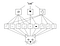Figure 14: Image patterns that activate neurons in different convolutional layers of a CNN show a hierarchy of increasing complexity: simple patterns (like edges) combine into local objects (like eyes or ears), which combine into higher-level concepts (such as a cat). Figure used with permission from Deep Learning with Python (Manning, 2017).

The number of convolutional layers and the numbers of kernels within each layer are tuning parameters in a CNN. When the complexity of the problem increases, you usually need more convolutional layers and more kernels per layer. In the last convolution layer of the CNN, we’ve a new representation of the input. Flattening all neurons of this layer into a vector results in a new feature representation of the image with as many features as were neurons in the last convolutional layer. We end up with the same situation as before: the input is described by a vector of image features, but this time the features are results from trained kernels. Now you can add a couple of densely connected layers to construct the prediction.Figure 15: A CNN with three convolutional layers followed by three fully connected layers. The number of feature maps in each convolutional layer indicate the number of kernel sets learned. The number of elements in each layer of the fully connected part indicate the number of weight sets learned.

Let’s try a CNN on the MNIST data. In listing 6, you see the definition of a CNN with convolutional layers followed by fully connected layers.

Open again the MNIST notebook https://github.com/tensorchiefs/dl_book/blob/master/chapter_02/nb_ch02_02.ipynb and fit a CNN with two convolutional layers to the MNIST data (see second part of the notebook) and compare the performance to what you achieved with an fcNN. Play with the code and perform a permutation experiment to check that the order of the pixels within the images matter for the performance of the CNN.

Listing 6. A CNN for MNIST classification

`# define CNN with two convolution blocks and two fully-connected layers model = Sequential()   model.add(Convolution2D(8,kernel_size,padding='same',input_shape=input_shape)) #A model.add(Activation('relu')) #B model.add(Convolution2D(8, kernel_size,padding='same')) #A model.add(Activation('relu')) #B model.add(MaxPooling2D(pool_size=pool_size)) #C   model.add(Convolution2D(16, kernel_size,padding='same')) #D model.add(Activation('relu')) #B model.add(Convolution2D(16,kernel_size,padding='same')) #D model.add(Activation('relu')) #B model.add(MaxPooling2D(pool_size=pool_size)) #E   model.add(Flatten())#F model.add(Dense(40))#G model.add(Activation('relu')) #D model.add(Dense(nb_classes))#G model.add(Activation('softmax'))#H   # compile model and initialize weights model.compile(loss='categorical_crossentropy',               optimizer='adam',               metrics=['accuracy'])   # train the model history=model.fit(X_train, Y_train,                   batch_size=128,                   epochs=10,                   verbose=2,                   validation_data=(X_val, Y_val)                  )`

#A Uses a convolutional layer with eight kernels of the size 3 x 3

#B Applies the relu activation function to the feature maps

#C This maxpooling layer has a pooling size of 2 x 2 and a stride of two

#D Uses a convolutional layer with sixteen kernels of the size 3 x 3

#E This maxpooling layer transforms the 14 x 14 x 16 input tensor to a 7 x 7 x 16 output tensor

#F Flattens the output of the previous layer resulting in a vector of length 784 (7*7*16)

#G Results into nb_classes (here ten) outputs

#H Uses the softmax to transform the ten outputs to ten prediction probabilities

The first convolutional layer with eight with the same padding results in an output feature maps which have the same size as the input image. In the MNIST case, the input image has a size 28 x 28 x 1 pixels, the resulting eight feature maps have a size of 28 x 28 x 8. After the first pooling the input has a shape of 28 x 28 x 8, and the output has a shape of 14 x 14 x 8.

From your experiments with the MNIST notebook https://github.com/tensorchiefs/dl_book/blob/master/chapter_02/nb_ch02_02.ipynb, you’ve learned that with this image classification task, it’s easy to achieve a higher performance with a CNN (around 99%) than with an fcNN (around 96%). The permutation experiment shows that the arrangement of the pixels within the image matters: the CNN performs much better when trained on the original image data (99%) than when trained on a shuffled version of the image data (95%). This supports the idea that the secret of the high performance of a CNN in image-related tasks lies in the architecture that takes the local order of an image into account.

Before wrapping up, let us look back and emphasize some advantages of CNNs when working with image data:

• Local connectivity makes use of the local information of image data.
• You need less weight parameters in a CNN than in an fcNN.
• A CNN is to a large extent invariant to translations within the images.
• The convolutional part of a CNN allows the network to learn hierarchically task-specific abstract image features

That’s all for now. If you want to learn more about the book, check it out on liveBook here and see this slide deck.

 People in the field of DL and computer vision use the word kernel, but sometimes you can also see the term filter which can be used as a synonym